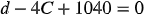1
57
views
18c
Problem

Problem 18c

Chapter 1: Functions and ModelsTextbook ExpertVerified Tutor
21 Oct 2021

Given information

Consider distance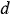on the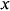axis and cost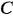on the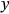axis.

Given,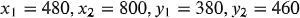Step-by-step explanation

Step 1.

The slope of the linear equation can be found by,The points in the form of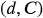are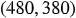and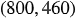The equation of the line was found to be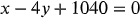or